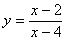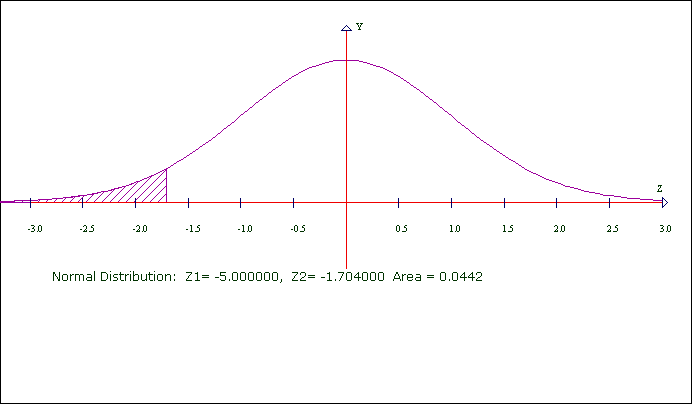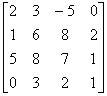# Homework

What follows is a (pretend) homework assignment, done using CalGraph.

Topics in Mathematics

Homework 1

1) Sketch the graph ofShow the vertical and horizontal asymptotes.

Solution:2) Sketch a graph showing these data and the straight line that best fits them.

Time       10       13        19        23         28

Money    \$50    \$84    \$139    \$185     \$225

What is the equation of that line ?

Solution:The line is   y =  9.7757 x   -  45.2298

3) The Over Sixties club admits some younger members so many that some people claim it is just a club and should not receive senior subsidies. The club claims its average age is 60 or more. Test this claim using alpha = 5% and this data: a random sample of 50 members had an average age of 58 with a standard deviation of 8.3.

Solution: 50 is more than 30, so the mean has a Normal Distribution. The z-score isThe area 0.0442 = 4.42% is less than 5%.

We fail to reject the null hypothesis.

We do not have enough evidence to say the club members average under 60.

4) Calculate the inverse ofSolution:How I did it:

In question 1, the formula was made with the Equation Editor in Microsoft Word. (Also in questions 3 and 4).

My first equation was  y = (x - 2)/(x - 4).  The second equation was  y = 1 (the horizontal asymptote).

To center the graph better, under Customize > Curve Domains/Range, I changed the Xmax to 10.

To do question 2, I opened Microsoft Excel. In the first column I put 10, 13, 19, 23 and 28.

In the second column I put 50, 84, 139, 185 and 225. I saved the spreadsheet as Homework.xls and closed Excel.

In CalGraph, I clicked Linear Algebra > Polynomial Curve Fitting. I put Homework.xls in the Excel file box, and listed the Top Left as A  1  and the Bottom Right as B  5. A straight line has degree 1, so I put a 1 in Degree.

I clicked Sketch and it drew a graph. To make it pretty a bit, under Customize > Curve Domains/Range I changed the Xmin to 0 and the Xmax to 30. For the same reason I changed the Y-min to 0 and the Ymax to 250. I used Text to name the graph Time versus Money.

The formula for the line was in the first box of CalGraph.

For question 3, I used the Statistics > Normal Distribution. I hit Edit > Copy Graph to Clipboard and Pasted into this document.

Question 4 was done using Linear Algebra > Matrices. I entered the matrix and then hit the Inverse button. Then I clicked Edit > Copy Matrices to Clipboard and pasted into this document.